# Anticipating your Opponent with Minimax Search

From Deep Learning and the Game of Go by Max Pumperla and Kevin Ferguson

___________________________________________________________________

Save 37% off Deep Learning and the Game of Go. Just enter fccpumperla into the discount code box at checkout at manning.com.
___________________________________________________________________

How can we program a computer to decide what move to make next in a game? To start, we can think about how humans would make the same decision. Let’s start with the simplest deterministic perfect information game there is: tic-tac-toe. The technical name for the strategy we’ll describe is minimaxing. “Minimaxing” is a contraction of “minimizing and maximizing”: you are trying to maximize your score, while your opponent is trying to minimize your score. You can sum it up the algorithm in one sentence: assume your opponent is as smart as you are. Let’s see how minimaxing works in practice.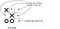Figure 1. What move should X make next? This is an easy one: playing in the lower right corner wins the game.

Take a look at figure 1. What move should X make next? There’s no trick here; taking the lower right corner wins the game. We can make that into a general rule: take any move that immediately wins the game. There’s no way this plan can go wrong. We could implement this rule in code with something like this:

`def find_winning_move(game_state, next_player):      for candidate_move in game_state.legal_moves(next_player):      ❶          next_state = game_state.apply_move(candidate_move)            ❷          if next_state.is_over() and next_state.winner == next_player:              return candidate_move                                       ❸      return None                                                     ❹`

❶ Loop over all legal moves

❷ Calculates what the board would look like if we pick this move

❸ This is a winning move! No need to continue searching

❹ Can’t win on this turn

Figure 2 illustrates the hypothetical board positions this function would examine. This structure, where a board position points to possible follow-ups, is called a game tree.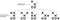Figure 2. An illustration of a algorithm to find the winning move. We start with the position at the top. We loop over every possible move and calculate the game state that would result if we played that move. Then we check if that hypothetical game state is a winning position for X.

Let’s back up a bit. How did we get into this position? Perhaps the previous position looked like figure 3. The O player naively hoped to make three in a row across the bottom. But that assumes that X will cooperate with the plan. This gives a corollary to our previous rule: don’t choose any move that gives our opponent a winning move.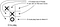Figure 3. What move should O make next? If O plays in the lower left, we must assume that X will follow up in the lower right to win the game. O must find the only move that prevents this.
`def eliminate_losing_moves(game_state, next_player):      opponent = next_player.other()      possible_moves = []                                              ❶        for candidate_move in game_state.legal_moves(next_player):       ❷          next_state = game_state.apply_move(candidate_move)             ❸          opponent_winning_move = find_winning_move(next_state, opponent)❹          if opponent_winning_move is None:                              ❹              possible_moves.append(candidate_move)                        ❹      return possible_moves`

❶ possible_moves will become a list of all moves worth considering

❷ Loops over all legal moves

❸ Calculates what the board would look like if we play this move

❹ Does this give our opponent a winning move? If not, this move is plausible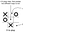Figure 4. What move should X make? If X plays in the center, then there will be two different ways to complete three-in-a-row: top middle and lower right. O can only block one of them, so X is guaranteed a win.

Now, we know that we must block our opponent from getting into a winning position. Therefore we should assume that our opponent is going to do the same to us. With that in mind, how can we play to win? Take a look at the board in figure 4. If we play in the center, we have two ways to complete three in a row: top middle or lower right. The opponent can’t block them both. We can describe this general principle as: look for a move where our opponent can’t block from setting up a winning move. Sounds complicated, but it’s actually easy to build this logic on top of the functions we’ve already written:

`def find_two_step_win(game_state, next_player):      opponent = next_player.other()      for candidate_move in game_state.legal_moves(next_player):      ❶          next_state = game_state.apply_move(candidate_move)            ❷          good_responses = eliminate_losing_moves(next_state, opponent) ❸          if not good_responses:                                        ❸              return candidate_move                                       ❸      return None                                                     ❹`

❶ Loop over all legal moves

❷ Calculates what the board would look like if we play this move

❸ Does our opponent have a good defense? If not, pick this move

❹ No matter what move we pick, our opponent can prevent a win

Of course, our opponent will anticipate that we will try to do this, and also try to block such a play. We can start to see a general strategy forming:

1. First, see if we can win on the next move. If so, play that move.
2. If not, see if our opponent can win on the next move. If so, block that.
3. If not, see if we can force a win in two moves. If so, play to set that up.
4. If not, see if our opponent could set up a two-move win on their next move…

Notice that all three of our functions have a similar structure. Each function loops over all valid moves and examines the hypothetical board position that we’d get after playing that move. Furthermore, each function builds on the previous function to simulate what our opponent would do in response. If we generalize this concept, we get an algorithm that can always identify the best possible move.

## Solving tic-tac-toe: a minimax example

In the previous section, we examined how to anticipate your opponent’s play one or two moves ahead. Here we show how to generalize that strategy to pick perfect moves in tic-tac-toe. The core idea is exactly the same, but we need the flexibility to look an arbitrary number of moves in the future.

First let’s define an enum that represents the three possible outcomes of a game: win, loss, or draw. These possibilities are defined relative to a particular player: a loss for one player is a win for the other.

Listing 1. An enum to represent the outcome of a game.

`class GameResult(enum.Enum):          loss = 1          draw = 2          win = 3`

Imagine we had a function `best_result` that took a game state and told us the best outcome that a player could achieve from that state. If that player could guarantee a win—by any sequence, no matter how complicated—the `best_result` function would return `GameResult.win`. If that player could force a draw, it would return `GameResult.draw`. Otherwise, it would return `GameResult.loss`. If we assume that function already exists, it’s easy to write a function to pick a move: we loop over all possible moves, call `best_result`, and pick the move that leads to the best result for us. Of course, there may be multiple moves that lead to equal results; we can just pick randomly from them in that case. Listing 2 shows how to implement this.

Listing 2. A game-playing agent that implements minimax search.

`class MinimaxAgent(Agent):          def select_move(self, game_state):                  winning_moves = []                  draw_moves = []                  losing_moves = []                  for possible_move in game_state.legal_moves():             ❶                          next_state = game_state.apply_move(possible_move)      ❷                          opponent_best_outcome = best_result(next_state)        ❸                          our_best_outcome = reverse_game_result(opponent_best_outcome)                         ❸                          if our_best_outcome == GameResult.win:                 ❹                                  winning_moves.append(possible_move)                ❹                                   elif our_best_outcome == GameResult.draw:              ❹                                  draw_moves.append(possible_move)                   ❹                          else:                                                  ❹                                  losing_moves.append(possible_move)                 ❹                       if winning_moves:                                      ❺                              return random.choice(winning_moves)                ❺                   if draw_moves:                                         ❺                           return random.choice(draw_moves)                   ❺                   return random.choice(losing_moves)                     ❺`

❶ Loops over all legal moves

❷ Calculates the game state if we select this move

❸ Since our opponent plays next, figure out their best possible outcome from there. Our outcome is the opposite of that

❹ Categorizes this move according to its outcome

❺ Picks a move that leads to our best outcome

Now the question is how to implement `best_result`. As in the previous section, we can start from the end of the game and work backward. Listing 3 shows the easy case: if the game is already over, there’s only one possible result. We just return it.

Listing 3. First step of the minimax search algorithm. If the game is already over, we already know the result.

`def best_result(game_state):    """Find the best result that next_player can get from this game    state.      Returns:        GameResult.win if next_player can guarantee a win        GameResult.draw if next_player can guarantee a draw        GameResult.loss if, no matter what next_player chooses, the           opponent can still force a win    """    if game_state.is_over():        if game_state.winner() == game_state.next_player:             return GameResult.win                 elif game_state.winner() is None:             return GameResult.draw        else:             return GameResult.loss`

If we’re somewhere in the middle of the game, we need to search ahead. By now, the pattern should be familiar. We start by looping over all possible moves and calculating the next game state. Then we must assume our opponent will do their best to counter our hypothetical move. To do so, we can just call `best_result` from this new position. That tells us the result our opponent can get from the new position; we invert it to find out our result. Out of all the moves we consider, we select the one that leads to the best result for us. Listing 4 shows how to implement this logic, which makes up the second half of `best_result`. Figure 5 illustrates the board positions this function will consider for a particular tic-tac-toe board.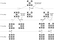Figure 5. A tic-tac-toe game tree. In the top position, it is X’s turn. If X plays in the top center, then O can guarantee a win. If X plays in the left center, X will win. If X plays right center, then O can force a draw. Therefore X will choose to play in the left center.

Listing 4.4. Implementing minimax search.

`best_result_so_far = GameResult.loss    opponent = game_state.next_player.other    for candidate_move in game_state.legal_moves():            ❶       next_state = game_state.apply_move(candidate_move)      ❷       opponent_best_result = best_result(next_state)          ❸       our_result = reverse_game_result(opponent_best_result)  ❹       if our_result.value > best_result_so_far.value:           best_result_so_far = our_result   return best_result_so_far`

❶ See what the board would look like if we play this move.

❷ Find out our opponent’s best move.

❸ Whatever our opponent wants, we want the opposite.

❹ See if this result is better than the best we’ve seen so far.

If we apply this algorithm to a simple game such as tic-tac-toe, we get an unbeatable opponent. You can play against it and see for yourself: try the `play_ttt.py` example on GitHub (https://github.com/maxpumperla/deep_learning_and_the_game_of_go). In theory, this algorithm would also work for chess, Go, or any other deterministic perfect information game. In reality, it’s far too slow for any of those games.

That’s all for now. If you want to learn more about the book, check it out on liveBook here and see this slide deck.

Max Pumperla and Kevin Ferguson are experienced deep learning specialists skilled in distributed systems and data science. Together, Max and Kevin built the open source bot BetaGo.

Originally published at freecontent.manning.com.

Written by